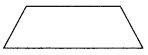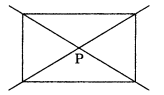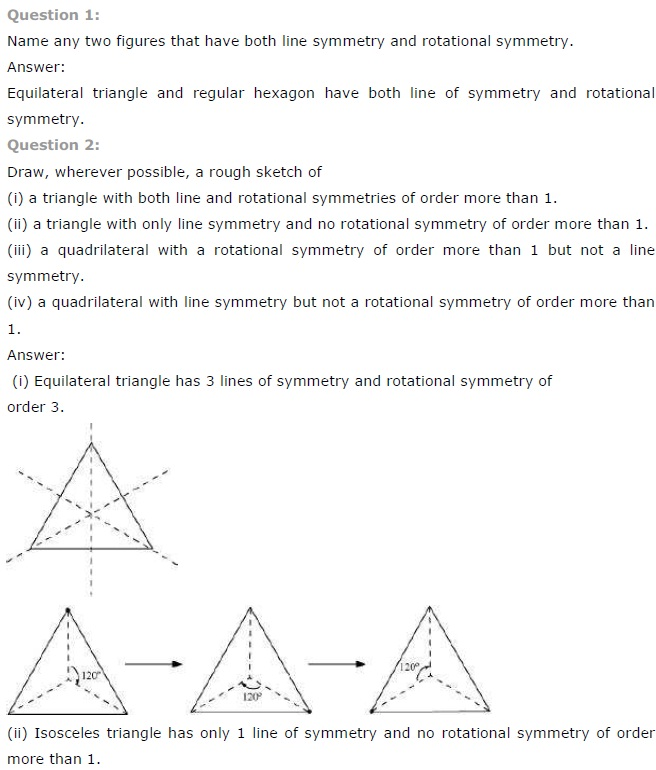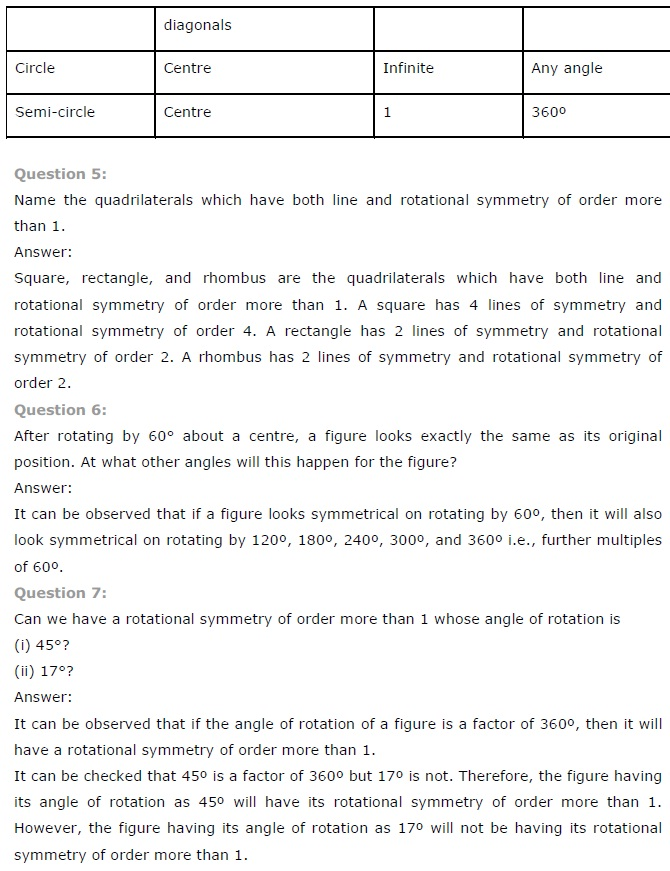# NCERT Solutions for Class 7 Maths Chapter 14 Symmetry Ex 14.3

NCERT Solutions for Class 7 Maths Chapter 14 Symmetry Ex 14.3

### NCERT Solutions for Class 7 Maths Chapter 14 Symmetry Ex 14.3

NCERT Solutions for Class 7 Maths Chapter 14 Symmetry Exercise 14.3
Ex 14.1 Class 7 Maths Question 1.
Name any two figures that have both line symmetry and rotational symmetry.
Solution:
English alphabet H and O both have line symmetry and rotational symmetry.

Ex 14.3 Class 7 Maths Question 2.
Draw, wherever possible, a rough sketch of
(i) a triangle with both line and rotational symmetries of order more than 1.
(ii) a triangle with only line symmetry and no rotational symmetry of order more than 1.

(iii) a quadrilateral with a rotational symmetry of order more than 1 but not a line symmetry.

(iv) a quadrilateral with line symmetry but not a rotational symmetry of order more than 1.
Solution:
(i) Equilateral triangle has 3 rotational symmetries.(ii) Not possible.
(iii)(iv) Not possible.

Ex 14.3 Class 7 Maths Question 3.
If a figure has two or more lines of symmetry, should it have rotational symmetry of order more than 1?
Solution:Yes. The above figure has two lines of symmetry and also rotational symmetry of order 2.

Ex 14.3 Class 7 Maths Question 4.
Fill in the blanks:

 Shape Centre of rotation Order of rotation Angle of rotation Square Rectangle Rhombus Equilateral triangle Regular hexagon Circle Semicircle

Solution:

 Shape Centre of rotation Order of rotation Angle of rotation Square Point of intersection of diagonals 4 90° Rectangle Point of intersection of diagonals 4 90° Rhombus Point of intersection of diagonals 4 90° Equilateral triangle Point of intersection of medians 3 120° Regular hexagon Point of intersection of diagonals 6 60° Circle Centre Infinite Every angle Semicircle Centre 4 90°

Ex 14.3 Class 7 Maths Question 5.
Name the quadrilaterals which have both line and rotational symmetry of order more than 1.
Solution:
Square, rectangles and rhombus are such quadrilateral which have both line and rotational symmetry.

Ex 14.3 Class 7 Maths Question 6.
After rotating by 60° about a centre, a figure looks exactly the same as its original position. At what other angles will this happen for the figure?
Solution:
If a figure is rotated through the angles 120°, 180°, 240°, 300° and 360°, it looks exactly the same.

Ex 14.3 Class 7 Maths Question 7.

Can we have a rotational symmetry of order more than 1 whose angle of rotation is
(i) 45°?
(ii) 17°?
Solution:
(i) Yes
(ii) No## SabDekho

The Complete Educational Website